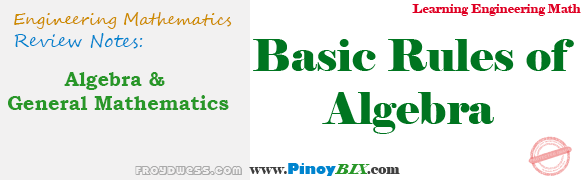# Algebra and General Mathematics: Basic Rules of Algebra

(Last Updated On: February 5, 2020)#### PROPERTIES OF REAL NUMBERS

1. Closure Property

Multiplication          :      a · b

2. Commutative Property

Addition          :      a + b = b + a
:      4m + n2 = n2 + 4m
Multiplication          :      ab = ba
:      (m – 3)n2 = n2(m – 3)

Addition          :      (a + b) + c = a + (b + c)
:      (m + 12) + 3n2 = m + (12 + 3n2)
Multiplication          :      (ab)c = a(bc)
:      (4m · 3n)6 = 4m(3n · 6)

4. Distributive Property

Right Distributive          :      a(b + c) = ab + ac
:     n(m + 9) = mn + 9n
Left Distributive          :      (a + b)c = ac + bc
:      (m + 9)n = mn + 9n

a + 0 = a           :      4m2 + 0 = 4m2

6. Multiplicative Identity Property

a · 1 = 1 · a = a           :      (5m2)(1) = (1)(5m2) = 5m2

a + (-a) = 0           :      7m2 + (-7m2) = 0

8. Multiplicative Inverse Property

a · 1/a = 1,  a ≠ 0           :      (3m2 + 4) [1 / (3m2 + 4)] = 1

#### PROPERTIES OF EQUALITY

1. Reflexive Property

a = a

2. Symmetric Property

If a = b, then b = a

3. Transitive Property

If a = b, and b = c, then a = c

If a = b, then a + c = b + c

5. Subtraction Property

If a = b, then a – c = = b – c

6. Substitution Property

If a = b, then a can be replaced by b in any expression involving a.

7. Multiplicative Property

If a = b, then ac = bc

8. Division Property

If a = b, then a/c = b/c, with c ≠ 0

9. Cancellation Property

If a + c = b + c, then a = b
If ac = bc, then a = b, provided c ≠ 0

#### OPERATIONS WITH ZERO AND INFINITY

1. a + 0 = a
2. a – 0 = a
3. a(0) = 0
4. 0/a = 0
5. a/∞ = 0
6. 0a = 0
7. a(∞) =
8. a/0 = undefined
9. ∞/a = ∞
10. a0 = 1, a ≠ 0

P inoyBIX educates thousands of reviewers and students a day in preparation for their board examinations. Also provides professionals with materials for their lectures and practice exams. Help me go forward with the same spirit.

“Will you subscribe today via YOUTUBE?”

Subscribe

• Full Content Access Exclusive to Premium members

## PINOYBIX FREEBIES FOR PREMIUM MEMBERSHIP:

• CIVIL ENGINEERING REVIEWER
• CIVIL SERVICE EXAM REVIEWER
• CRIMINOLOGY REVIEWER
• ELECTRONICS ENGINEERING REVIEWER (ECE/ECT)
• ELECTRICAL ENGINEERING & RME REVIEWER
• FIRE OFFICER EXAMINATION REVIEWER
• LET REVIEWER
• MASTER PLUMBER REVIEWER
• MECHANICAL ENGINEERING REVIEWER
• NAPOLCOM REVIEWER

## FOR A LIMITED TIME

If you subscribe for PREMIUM today!